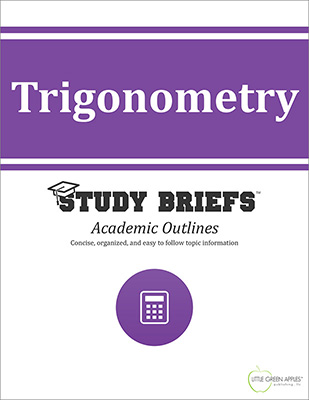# TrigonometryLook inside
• Format: eBook
• Edition: 2016
• Publisher: Little Green Apples Publishing LLC

Price: \$4.99

## Description:

Need help with your Trig homework? Could you benefit from having quick access to fundamental facts and formulas? The Trigonometry Study Brief is intended as a quick and convenient reference for students enrolled in a basic trigonometry class. It is ideal for any adult learner approaching the study of advanced mathematics who may benefit from a quick refresher on foundational trigonometric topics. This academic outline collects together a wealth of useful examples, formulas and equations in a highly visual format. The focus is to highlight key concepts, theorems, laws and principles needed to understand advanced trigonometric functions. Numerous examples with clear explanations should be helpful guides when working through your own problems involving complex numbers. Detailed sections on these topics are included: formulas such as the Distance Formula; theorems such as the Pythagorean Theorem; variables; angles; triangles and vectors; the Law of Sines and the Law of Cosines; how to use a Trigonometric Table; radian measures and circular functions; horizontal translations, phase shifts, and simple harmonic motion; trigonometric identities; inverse trigonometric functions; trigonometric equations; complex numbers, including absolute values, DeMoives' Theorum and polar equations; exponential functions and equations; and logarithmic functions and equations.

## Topics Covered:

• Trigonometric Functions
• Acute Angles and Right Triangles
• Radian Measure and Circular Functions
• Trigonometric Identities
• Inverse Trigonometric Function and Trigonometric Equations
• Triangles and Vectors
• Complex Numbers
• Logarithms
• References

Browse Subjects